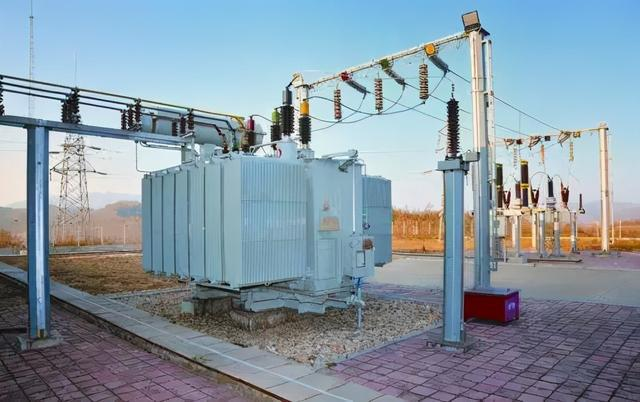# How much kW of power consumption can a 1000kVA transformer withstand?

November 12, 2021

There is an old-fashioned 1000kVA transformer with an existing load of about 200kW. If a new load of about 600kW is to be added, can this transformer bear it?The capacity unit of the transformer is kVA (kilovolt-ampere), while the power unit of the electrical equipment is kW (kilowatt). The difference between the two is that the power factor needs to be multiplied when calculating the power kW of the equipment, that is, a transformer with a capacity of 1000kVA. Only when the power factor is 1, it can output 1000kW of power at full load, but it is basically impossible in practical applications.

When designing, it is necessary to leave a certain margin, which is generally calculated according to a 90% coincidence rate, which is more economical and reasonable, that is, 1000×0.9=900kVA. If we compensate the power factor to 0.95 and above through power compensation, then the transformer can output 900×0.95=855kW active power.
Therefore, the 1000kVA transformer originally supplied power to the 200kW electrical equipment, and now 600kW electrical equipment has been added. The total active power of the electrical equipment has reached 800kW, which still does not exceed the calculated value.